###### eISSN: 2378-315X# Biometrics & Biostatistics International JournalResearch Article Volume 7 Issue 6

# Modeling lifetime data with Weibull-Lindley distribution

#### Ieren TG, function clickButton(){ var name=document.getElementById('name').value; var descr=document.getElementById('descr').value; var unCopyslNo=document.getElementById('unCopyslNo').value; document.getElementById("mydiv").style.display = "none"; $.ajax({ type:"post", url:"https://medcraveonline.com/captchaCode/server_action", data: { 'name' :name, 'descr' :descr, 'unCopyslNo': unCopyslNo }, cache:false, success: function (html) { //alert('Data Send');$('#msg').html(html); } }); return false; } Verify Captcha Regret for the inconvenience: we are taking measures to prevent fraudulent form submissions by extractors and page crawlers. Please type the correct Captcha word to see email ID.function refreshPage(){ $("#mydiv").load(location.href + " #mydiv"); }$(document).ready(function () { //Disable cut copy paste $('#msg').bind('cut copy paste', function (e) { e.preventDefault(); }); //Disable mouse right click$("#msg").on("contextmenu",function(e){ return false; }); }); .noselect { -webkit-touch-callout: none; /* iOS Safari */ -webkit-user-select: none; /* Safari */ -khtml-user-select: none; /* Konqueror HTML */ -moz-user-select: none; /* Firefox */ -ms-user-select: none; /* Internet Explorer/Edge */ user-select: none; /* Non-prefixed version, currently supported by Chrome and Opera */ cursor: none; } Oyamakin SO, Chukwu AU

Department of Statistics, University of Ibadan, Nigeria

Received: October 15, 2018 | Published: November 23, 2018

citation: Ieren TG, Oyamakin SO, Chukwu AU. Modeling lifetime data with Weibull-Lindley distribution. Biom Biostat Int J. 2018;7(6):532–544. DOI: 10.15406/bbij.2018.07.00256

# Abstract

In this paper a new extension of the Lindley distribution is presented using the Weibull link function introduced and studied by Tahir et al.,1 to develop a Weibull-Lindley distribution. We derive and discuss the mathematical and Statistical properties of the subject distribution along with its reliability analysis and inference for the parameters. Finally, the Weibull-Lindley distribution has been used to model four lifetime datasets and the results show that the proposed generalization performs better than the other known extensions of the Lindley distribution considered for the study.

Keywords: Lindley distribution, Weibull-Lindley distribution, mathematical properties, reliability function, parameter estimation, applications.

# Introduction

The Lindley distribution introduced by Lindley et al.,2 in the context of Bayesian analysis as a counter example of fiducial statistics, is defined by its probability density function (PDF) and cumulative distribution function (CDF) as

$G\left(x\right)=1-\left[1+\frac{\theta x}{\theta +1}\right]{e}^{-\theta x}$ (1.1)

And

$g\left(x\right)=\frac{{\theta }^{2}}{\theta +1}\left(1+x\right){e}^{-\theta x}$ (1.2)

respectively. For $x>0,\theta >0,$ where $\theta$ is the scale parameter of the Lindley distribution.

Details of this distribution, its mathematical and statistical properties, estimation of its parameter and application including the superiority of Lindley distribution over exponential distribution has been done by Ghitany et al.,3 We have so many generalized families of distributions proposed by different researchers that are used in extending other distributions to produce compound distributions with better performance. These are several ways of adding one or more parameters to a distribution function which makes the resulting distribution richer and more flexible for modeling data. A brief summary of some of these methods or families of distribution include the beta generalized family (Beta-G) by Eugene et al.,4 the Kumaraswamy-G by Cordeiro et al.5  Transmuted family of distributions by Shaw et al.,6 Gamma-G (type 1) by Zografos et al.,7 McDonald-G by Alexander et al.,8 Gamma-G (type 2) by Risti et al.,9 Gamma-G (type 3) by Torabi et al.,10 Log-gamma-G by Amini et al.,9 Exponentiated T-X by Alzaghal et al.,12 Exponentiated-G (EG) by Cordeiro et al.,13 Logistic-G by Torabi et al.,14 Gamma-X by Alzaatreh et al.,15 Logistic-X by Tahir et al.,16 Weibull-X by Alzaatreh et al.,17 Weibull-G by Bourguignon et al.,18 a new Weibull-G family by Tahir et al.,1 a Lomax-G family by Cordeiro et al.,19 a new generalized Weibull-G family by Cordeiro et al.,20 and Beta Marshall-Olkin family of distributions by Alizadeh et al.,21 and some other families of the distributions.

Hence, there are also some generalizations of the Lindley distribution recently proposed in the literature such as the transmuted Lindley distribution by Merovci et al.,23 the exponentiated Power Lindley distribution by Ashour et al.,24 Generalized Lindley distribution by Nadarajah et al.,24 Transmuted Generalized Lindley distribution by Elgarhy et al.,25 Extended Power Lindley distribution by Alkarni et al.,26 a two-parameter Lindley distribution by Shanker et al.,27 the Lomax-Lindley distribution by Yahaya et al.,28 Transmuted Two-Parameter Lindley distribution by Al-khazaleh et al.,29 and a three-parameter Lindley distribution by Shanker et al.,30 The aim of this article is to introduce a new continuous distribution called Weibull-Lindley distribution (WLnD) from the proposed family by Tahir et al.,1 The remaining parts of this article are presented in sections as follows: We defined the new distribution and give its plots in section 2.1. Section 2.2 derived some properties of the new distribution. Section 2.3 proposes some reliability functions of the new distribution. The order statistics for the new distribution are also given in section 2.4. The maximum likelihood estimates (MLEs) of the unknown model parameters of the new distribution are obtained in section 2.5. In section 3 we carryout application of the proposed model with others to four lifetime datasets. Lastly, in section 4, we give the summary of our work and concluding remarks.

# Materials and methods

Construction of Weibull-Lindley distribution (WLnD)

In the next section, we have defined the cdf and pdf of the Weibull-Lindley distribution (WLnD) using the method proposed by Tahir et al.1 According to Tahir et al.,1  the formula or Weibull link function for deriving the cdf and pdf of any Weibull-based continuous distribution is defined as:

$F\left(x\right)=\underset{0}{\overset{-\mathrm{log}\left[G\left(x\right)\right]}{\int }}\alpha \beta {t}^{\beta -1}{e}^{-\alpha {t}^{\beta }}dt={e}^{-\alpha {\left\{-\mathrm{log}\left[G\left(x\right)\right]\right\}}^{\beta }}$  (2.1.1)

And

$f\left(x\right)=\alpha \beta \frac{g\left(x\right)}{G\left(x\right)}{\left\{-\mathrm{log}\left[G\left(x\right)\right]\right\}}^{\beta -1}{e}^{-\alpha {\left\{-\mathrm{log}\left[G\left(x\right)\right]\right\}}^{\beta }}$  (2.1.2)

respectively, where $g\left(x\right)$  and $G\left(x\right)$  are the pdf and cdf of any continuous distribution to be generalized respectively and $\alpha$  and $\beta$  are the two additional new parameters responsible for the shape of the distribution.

Using equation (1.1) and (1.2) in (2.1.1) and (2.1.2) and simplifying, we obtain the cdf and pdf of the Weibull-Lindley random variable X as:

$F\left(x;\theta ,\alpha ,\beta \right)={e}^{-\alpha {\left\{-\mathrm{log}\left[1-\left[1+\frac{\theta x}{\theta +1}\right]{e}^{-\theta x}\right]\right\}}^{\beta }}$  (2.1.3)

And

$f\left(x;\theta ,\alpha ,\beta \right)=\frac{\alpha \beta {\theta }^{2}\left(1+x\right){e}^{-\theta x}}{\left(\theta +1\right)\left[1-\left[1+\frac{\theta x}{\theta +1}\right]{e}^{-\theta x}\right]}{\left\{-\mathrm{log}\left[1-\left[1+\frac{\theta x}{\theta +1}\right]{e}^{-\theta x}\right]\right\}}^{\beta -1}{e}^{-\alpha {\left\{-\mathrm{log}\left[1-\left[1+\frac{\theta x}{\theta +1}\right]{e}^{-\theta x}\right]\right\}}^{\beta }}$  (2.1.4)

respectively.

For $x>0;\theta ,\alpha ,\beta >0$ ; where $\theta$  is a scale parameter and $\alpha$  and $\beta$  is a shape parameters of the Weibull-Lindley distribution.

The following is a graphical representation of the pdf and cdf of the Weibull-Lindley distribution. Given some values of the parameters $\alpha ,\beta &\theta$ , we provide some possible graphs for the pdf and the cdf of the WLnD as shown in Figure 1&2 below: Figure 1 indicates that the WLnD is a skewed distribution and can take various forms. This means that distribution can be very useful for datasets that are skewed.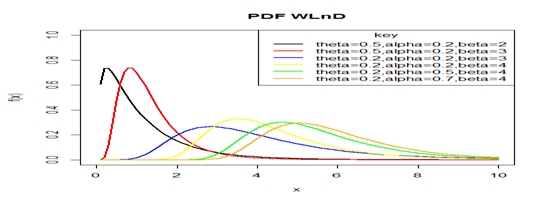Figure 1 Graph of PDF of the WLnD for varrying parameter values.Figure 2 Graph of CDF of the WLnD for varying parameter values.

From the above cdf plot, the cdf increases when X increases, and approaches 1 when X becomes large, as expected.

# Properties

In this section, we defined and discuss some properties of the WLnD distribution.

The Quantile function

This function is derived by inverting the cdf of any given continuous probability distribution. It is used for obtaining some moments like skewness and kurtosis as well as the median and for generation of random variables from the distribution in question. Hyndman et al.,21 defined the quantile function for any distribution in the form  Q(u) = ${F}^{-1}$ (u)  where Q(u) is the quantile function of F(x) for 0 < u <1

Taking F(x) to be the cdf of the Weibull-Lindley distribution and inverting it as above will give us the Quantile function as follows:

$F\left(x\right)={e}^{-\alpha {\left\{-\mathrm{log}\left[1-\left[1+\frac{\theta x}{\theta +1}\right]{e}^{-\theta x}\right]\right\}}^{\beta }}=u$  (2.2.1)

Simplifying equation (2.2.1) above, we obtain:

$Q\left(u\right)={X}_{q}=-1-\frac{1}{\theta }-\frac{1}{\theta }W\left(-\frac{\left(\theta +1\right)\left(1-\mathrm{exp}\left\{-{\left(-\frac{\mathrm{ln}u}{\alpha }\right)}^{\frac{1}{\alpha }}\right\}\right)}{{e}^{\theta +1}}\right)$  (2.2.2)

By using (2.2.2) above, the median of X from the WLnD is simply obtained by setting u=0.5 while random numbers can be generated from WLnD by setting $X=Q\left(u\right)$ , where u is a uniform variate on the unit interval (0,1) and $W\left(.\right)$ represents the negative branch of the Lambert function.

Skewness and kurtosis

The quantile based measures of skewness and kurtosis will employed due to non-existence of the classical measures in some cases. The Bowley’s measure of skewness based on quartiles by Kenney et al.,32 is given as;

< $SK=\frac{Q\left(\frac{3}{4}\right)-2Q\left(\frac{1}{2}\right)+Q\left(\frac{1}{4}\right)}{Q\left(\frac{3}{4}\right)-Q\left(\frac{1}{4}\right)}$  (2.2.3)

while the Risti et al.,9 kurtosis based on octiles is given by;

$KT=\frac{Q\left(\frac{7}{8}\right)-Q\left(\frac{5}{8}\right)-Q\left(\frac{3}{8}\right)+\left(\frac{1}{8}\right)}{Q\left(\frac{6}{8}\right)-Q\left(\frac{1}{4}\right)}$  (2.2.4)

where Q(.) is any quartile or octile of interest.

Moments

Moments of a random variable are very important in distribution theory because they are used to study some of the most important features and characteristics of a random variable such as mean, variance, skewness and kurtosis.

Let X denote a continuous random variable, the nth moment of X is given by;

${\mu }_{n}^{\text{'}}=E\left[{X}^{n}\right]=\underset{0}{\overset{\infty }{\int }}{x}^{n}f\left(x\right)dx$ (2.2.5)

Considering f(x) to be the pdf of the Weibull-Lindley distribution as given in equation (2.1.4)

${\mu }_{n}^{\text{'}}=E\left[{X}^{n}\right]=\underset{0}{\overset{1}{\int }}{x}^{n}f\left(x\right)dx$

Recall that from equation (2.1.4),

$f\left(x;\theta ,\alpha ,\beta \right)=\frac{\alpha \beta {\theta }^{2}\left(1+x\right){e}^{-\theta x}}{\left(\theta +1\right)\left[1-\left[1+\frac{\theta x}{\theta +1}\right]{e}^{-\theta x}\right]}{\left\{-\mathrm{log}\left[1-\left[1+\frac{\theta x}{\theta +1}\right]{e}^{-\theta x}\right]\right\}}^{\beta -1}{e}^{-\alpha {\left\{-\mathrm{log}\left[1-\left[1+\frac{\theta x}{\theta +1}\right]{e}^{-\theta x}\right]\right\}}^{\beta }}$  (2.2.6)

Let

$A={e}^{-\alpha {\left\{-\mathrm{log}\left[1-\left[1+\frac{\theta x}{\theta +1}\right]{e}^{-\theta x}\right]\right\}}^{\beta }}$

Then, using a power series expansion for A, we can write A as:

$A={e}^{-\alpha {\left\{-\mathrm{log}\left[1-\left[1+\frac{\theta x}{\theta +1}\right]{e}^{-\theta x}\right]\right\}}^{\beta }}=\sum _{i=0}^{\infty }\frac{{\left(-1\right)}^{i}{\alpha }^{i}}{i!}{\left(-\mathrm{log}\left[1-\left[1+\frac{\theta x}{\theta +1}\right]{e}^{-\theta x}\right]\right)}^{\beta i}$

Substituting for the expansion above in equation (2.2.6), we have;

$f\left(x\right)=\frac{\alpha \beta {\theta }^{2}\left(1+x\right){e}^{-\theta x}}{\left(\theta +1\right)\left[1-\left[1+\frac{\theta x}{\theta +1}\right]{e}^{-\theta x}\right]}{\left\{-\mathrm{log}\left[1-\left[1+\frac{\theta x}{\theta +1}\right]{e}^{-\theta x}\right]\right\}}^{\beta -1}\sum _{i=0}^{\infty }\frac{{\left(-1\right)}^{i}{\alpha }^{i}}{i!}{\left(-\mathrm{log}\left[1-\left[1+\frac{\theta x}{\theta +1}\right]{e}^{-\theta x}\right]\right)}^{\beta i}$

$f\left(x\right)=\frac{\alpha \beta {\theta }^{2}\left(1+x\right){e}^{-\theta x}}{\left(\theta +1\right)\left[1-\left[1+\frac{\theta x}{\theta +1}\right]{e}^{-\theta x}\right]}\sum _{i=0}^{\infty }\frac{{\left(-1\right)}^{i}{\alpha }^{i}}{i!}{\left(-\mathrm{log}\left[1-\left[1+\frac{\theta x}{\theta +1}\right]{e}^{-\theta x}\right]\right)}^{\beta \left(i+1\right)-1}$  (JKJK) (2.2.7)

Also, let

$B={\left(-\mathrm{log}\left[1-\left[1+\frac{\theta x}{\theta +1}\right]{e}^{-\theta x}\right]\right)}^{\beta \left(i+1\right)-1}$

Now, considering the following formula from Tahir et al.,1 which holds for B for i≥1, and then we can write B as follows:

${\left(-\mathrm{log}\left[1-\left[1+\frac{\theta x}{\theta +1}\right]{e}^{-\theta x}\right]\right)}^{\beta \left(i+1\right)-1}=\sum _{k,l=0}^{\infty }\sum _{j=0}^{k}\frac{{\left(-1\right)}^{j+k+l}\left(\beta \left(i+1\right)\right)}{\left(\beta \left(i+1\right)-1-j\right)}\left(\begin{array}{c}k-\left(\beta \left(i+1\right)-1\right)\\ k\end{array}\right)\left(\begin{array}{c}k\\ j\end{array}\right)\left(\begin{array}{c}\left(\beta \left(i+1\right)-1\right)+k\\ l\end{array}\right){P}_{j,k}{\left[1-\left[1+\frac{\theta x}{\theta +1}\right]{e}^{-\theta x}\right]}^{l}$  (2.2.8)

Where for (for j≥0) Pj,0=1 and (for k=1,2,…..)

${P}_{j,k}={k}^{-1}\sum _{m=1}^{k}{\left(-1\right)}^{m}\frac{\left[m\left(j+1\right)-k\right]}{\left(m+1\right)}{P}_{j,k-m}$  (2.2.9)

Combining equation (2.2.8) and (2.2.9) and inserting the above power series in equation (2.2.7) and simplifying, we have:

$f\left(x\right)=\frac{\alpha \beta {\theta }^{2}}{\left(\theta +1\right)}\sum _{i=0}^{\infty }\frac{{\left(-1\right)}^{i}{\alpha }^{i}}{i!}\sum _{k,l=0}^{\infty }\sum _{j=0}^{k}\frac{{\left(-1\right)}^{j+k+l}\left(\beta \left(i+1\right)\right)}{\left(\beta \left(i+1\right)-1-j\right)}\left(\begin{array}{c}k-\left(\beta \left(i+1\right)-1\right)\\ k\end{array}\right)\left(\begin{array}{c}k\\ j\end{array}\right)\left(\begin{array}{c}\left(\beta \left(i+1\right)-1\right)+k\\ l\end{array}\right){P}_{j,k}\left(1+x\right){e}^{-\theta x}{\left[1-\left[1+\frac{\theta x}{\theta +1}\right]{e}^{-\theta x}\right]}^{l-1}$

$f\left(x\right)=\frac{\alpha \beta {\theta }^{2}}{\left(\theta +1\right)}\sum _{i=0}^{\infty }\sum _{k,l=0}^{\infty }\sum _{j=0}^{k}\frac{{\left(-1\right)}^{i+j+k+l}{\alpha }^{i+1}\left(\beta \left(i+1\right)\right)}{i!\left(\beta \left(i+1\right)-1-j\right)}\left(\begin{array}{c}k-\left(\beta \left(i+1\right)-1\right)\\ k\end{array}\right)\left(\begin{array}{c}k\\ j\end{array}\right)\left(\begin{array}{c}\left(\beta \left(i+1\right)-1\right)+k\\ l\end{array}\right){P}_{j,k}\left(1+x\right){e}^{-\theta x}{\left[1-\left[1+\frac{\theta x}{\theta +1}\right]{e}^{-\theta x}\right]}^{l-1}$ (2.2.10)

Now,if l is a positive non-integer, we can expand the last term in (2.2.10) as:

${\left[1-\left[1+\frac{\theta x}{\theta +1}\right]{e}^{-\theta x}\right]}^{l-1}=\sum _{m=0}^{\infty }{\left(-1\right)}^{m}\left(\begin{array}{c}l-1\\ m\end{array}\right){\left[\left[1+\frac{\theta x}{\theta +1}\right]{e}^{-\theta x}\right]}^{m}$  (2.2.11)

Therefore, f(x) becomes:

$f\left(x\right)=\frac{\alpha \beta {\theta }^{2}}{\left(\theta +1\right)}\sum _{m=0}^{\infty }\sum _{i=0}^{\infty }\sum _{k,l=0}^{\infty }\sum _{j=0}^{k}\frac{{\left(-1\right)}^{i+j+k+l+m}{\alpha }^{i+1}\left(\beta \left(i+1\right)\right)}{i!\left(\beta \left(i+1\right)-1-j\right)}\left(\begin{array}{c}k-\left(\beta \left(i+1\right)-1\right)\\ k\end{array}\right)\left(\begin{array}{c}l-1\\ m\end{array}\right)\left(\begin{array}{c}k\\ j\end{array}\right)\left(\begin{array}{c}\left(\beta \left(i+1\right)-1\right)+k\\ l\end{array}\right){P}_{j,k}\left(1+x\right){e}^{-\theta x\left(1+m\right)}{\left[1+\frac{\theta x}{\theta +1}\right]}^{m}$  (2.2.12)

Using power series expansion on the last term in equation (2.2.12), we have

${\left(1+\frac{\theta x}{\theta +1}\right)}^{m}=\sum _{p=0}^{\infty }\left(\begin{array}{c}m\\ p\end{array}\right){\left(\frac{\theta x}{\theta +1}\right)}^{p}=\sum _{p=0}^{\infty }\left(\begin{array}{c}m\\ p\end{array}\right){\left(\frac{\theta }{\theta +1}\right)}^{p}{x}^{p}$  (2.2.13)

Now, substituting equation (2.2.13), the power series expansion in equation (2.2.12) above, one gets:

$f\left(x\right)=\frac{\alpha \beta {\theta }^{2}}{\left(\theta +1\right)}\sum _{p=0}^{\infty }\sum _{m=0}^{\infty }\sum _{i=0}^{\infty }\sum _{k,l=0}^{\infty }\sum _{j=0}^{k}\frac{{\left(-1\right)}^{i+j+k+l+m}{\alpha }^{i+1}\left(\beta \left(i+1\right)\right)}{i!\left(\beta \left(i+1\right)-1-j\right)}\left(\begin{array}{c}k-\left(\beta \left(i+1\right)-1\right)\\ k\end{array}\right)\left(\begin{array}{c}l-1\\ m\end{array}\right)\left(\begin{array}{c}m\\ p\end{array}\right){\left(\frac{\theta }{\theta +1}\right)}^{p}\left(\begin{array}{c}k\\ j\end{array}\right)\left(\begin{array}{c}\left(\beta \left(i+1\right)-1\right)+k\\ l\end{array}\right){P}_{j,k}\left(1+x\right){x}^{p}{e}^{-\theta x\left(1+m\right)}$

This implies that:

$f\left(x\right)={\eta }_{i,j,k,l,m,p}\left(1+x\right){x}^{p}{e}^{-\theta x\left(1+m\right)}$  (2.2.14)

Where

${\eta }_{i,j,k,l,m,p}=\frac{\alpha \beta {\theta }^{2}}{\left(\theta +1\right)}\sum _{p=0}^{\infty }\sum _{m=0}^{\infty }\sum _{i=0}^{\infty }\sum _{k,l=0}^{\infty }\sum _{j=0}^{k}\frac{{\left(-1\right)}^{i+j+k+l+m}{\alpha }^{i+1}\left(\beta \left(i+1\right)\right)}{i!\left(\beta \left(i+1\right)-1-j\right)}\left(\begin{array}{c}k-\left(\beta \left(i+1\right)-1\right)\\ k\end{array}\right)\left(\begin{array}{c}l-1\\ m\end{array}\right)\left(\begin{array}{c}m\\ p\end{array}\right){\left(\frac{\theta }{\theta +1}\right)}^{p}\left(\begin{array}{c}k\\ j\end{array}\right)\left(\begin{array}{c}\left(\beta \left(i+1\right)-1\right)+k\\ l\end{array}\right){P}_{j,k}$

Hence,

${\mu }_{n}^{\text{'}}=E\left[{X}^{n}\right]=\underset{0}{\overset{\infty }{\int }}{x}^{n}f\left(x\right)dx=\underset{0}{\overset{\infty }{\int }}{\eta }_{i,j,k,l,m,p}\left(1+x\right){x}^{n+p}{e}^{-\theta x\left(1+m\right)}dx$

${\mu }_{n}^{\text{'}}=E\left[{X}^{n}\right]=\underset{0}{\overset{\infty }{\int }}{x}^{n}f\left(x\right)dx={\eta }_{i,j,k,l,m,p}\left[\underset{0}{\overset{\infty }{\int }}{x}^{n+p}{e}^{-\theta x\left(1+m\right)}dx+\underset{0}{\overset{\infty }{\int }}{x}^{n+p+1}{e}^{-\theta x\left(1+m\right)}dx\right]$  (2.2.15)

Also, using integration by substitution method in equation (2.2.15); we obtain the following:

Let $u=\theta x\left(1+m\right)⇒x=\frac{u}{\theta \left(1+m\right)}$ ; $\frac{du}{dx}=\theta \left(1+m\right)$  and $dx=\frac{du}{\theta \left(1+m\right)}$

Substituting for $u$ , $x$ and $dx$  in equation (2.2.15) and simplifying; we have:

${\mu }_{n}^{\text{'}}={\eta }_{i,j,k,l,m,p}\left[\underset{0}{\overset{\infty }{\int }}{\left(\frac{u}{\theta \left(1+m\right)}\right)}^{n+p}{e}^{-u}\left(\frac{du}{\theta \left(1+m\right)}\right)+\underset{0}{\overset{\infty }{\int }}{\left(\frac{u}{\theta \left(1+m\right)}\right)}^{n+p+1}{e}^{-u}\left(\frac{du}{\theta \left(1+m\right)}\right)\right]$

${\mu }_{n}^{\text{'}}={\eta }_{i,j,k,l,m,p}\left[\frac{1}{{\left(\theta \left(1+m\right)\right)}^{n+p+1}}\underset{0}{\overset{\infty }{\int }}{u}^{n+p}{e}^{-u}du+\frac{1}{{\left(\theta \left(1+m\right)\right)}^{n+p+2}}\underset{0}{\overset{\infty }{\int }}{u}^{n+p+1}{e}^{-u}du\right]$

${\mu }_{n}^{\text{'}}={\eta }_{i,j,k,l,m,p}\left[\frac{1}{{\left(\theta \left(1+m\right)\right)}^{n+p+1}}\underset{0}{\overset{\infty }{\int }}{u}^{n+p+1-1}{e}^{-u}du+\frac{1}{{\left(\theta \left(1+m\right)\right)}^{n+p+2}}\underset{0}{\overset{\infty }{\int }}{u}^{n+p+2-1}{e}^{-u}du\right]$  (2.2.16)

Again recall that $\underset{0}{\overset{\infty }{\int }}{t}^{k-1}{e}^{-t}dt=\Gamma \left(k\right)$ and that $\underset{0}{\overset{\infty }{\int }}{t}^{k}{e}^{-t}dt=\underset{0}{\overset{\infty }{\int }}{t}^{k+1-1}{e}^{-t}dt=\Gamma \left(k+1\right)$

Thus we obtain the nth ordinary moment of X for the Weibull-Lindley distribution as follows:

${\mu }_{n}^{\text{'}}={\eta }_{i,j,k,l,m,p}\left[\frac{\Gamma \left(n+p+1\right)}{{\left(\theta \left(1+m\right)\right)}^{n+p+1}}+\frac{\Gamma \left(n+p+2\right)}{{\left(\theta \left(1+m\right)\right)}^{n+p+2}}\right]$  (2.2.17)

The Mean

The mean of the WLnD can be obtained from the nth moment of the distribution when n=1 as follows:

${\mu }_{n}^{\text{'}}={\eta }_{i,j,k,l,m,p}\left[\frac{\Gamma \left(n+p+1\right)}{{\left(\theta \left(1+m\right)\right)}^{n+p+1}}+\frac{\Gamma \left(n+p+2\right)}{{\left(\theta \left(1+m\right)\right)}^{n+p+2}}\right]$

$E\left[X\right]={\mu }_{1}^{\text{'}}={\eta }_{i,j,k,l,m,p}\left[\frac{\Gamma \left(p+2\right)}{{\left(\theta \left(1+m\right)\right)}^{p+2}}+\frac{\Gamma \left(p+3\right)}{{\left(\theta \left(1+m\right)\right)}^{p+3}}\right]$  (2.2.17)

The Variance

The nth central moment or moment about the mean of X, say ${\mu }_{n}$ , can be obtained as

${\mu }_{n}=E{\left[X-{\mu }_{1}^{\text{'}}\right]}^{n}=\sum _{i=0}^{n}{\left(-1\right)}^{i}\left(\begin{array}{c}n\\ i\end{array}\right){\mu }_{1}^{\text{'}i}{\mu }_{n-i}^{\text{'}}$  (2.2.18)

The variance of X for WLnD is obtained from the nth central moment when n=2, that is, the variance of X is the nth central moment of order two (n=2) and is given as follows:

$Var\left(X\right)=E\left[{X}^{2}\right]-{\left\{E\left[X\right]\right\}}^{2}$ (2.2.19)

$Var\left(X\right)={\mu }_{2}{}^{\text{'}}-{\left\{{\mu }_{1}{}^{\text{'}}\right\}}^{2}$

$Var\left(X\right)={\eta }_{i,j,k,l,m,p}\left[\frac{\Gamma \left(p+3\right)}{{\left(\theta \left(1+m\right)\right)}^{p+3}}+\frac{\Gamma \left(p+4\right)}{{\left(\theta \left(1+m\right)\right)}^{p+4}}\right]-{\left\{{\eta }_{i,j,k,l,m,p}\left[\frac{\Gamma \left(p+2\right)}{{\left(\theta \left(1+m\right)\right)}^{p+2}}+\frac{\Gamma \left(p+3\right)}{{\left(\theta \left(1+m\right)\right)}^{p+3}}\right]\right\}}^{2}$  (2.2.20)

The coefficients variation, skewness and kurtosis measures can also be calculated from the non-central moments using some well-known relationships.

Moment generating function

The mgf of a random variable X can be obtained by

${M}_{x}\left(t\right)=E\left[{e}^{tx}\right]=\underset{0}{\overset{\infty }{\int }}{e}^{tx}f\left(x\right)dx$  (2.2.21)

Using power series expansion in equation (2.2.21) and simplifying the integral therefore we have;

${M}_{x}\left(t\right)=\sum _{n=0}^{\infty }\frac{{t}^{n}}{n!}{\mu }_{n}^{\text{'}}=\sum _{n=0}^{\infty }\frac{{t}^{n}}{n!}\left\{{\eta }_{i,j,k,l,m,p}\left[\frac{\Gamma \left(n+p+1\right)}{{\left(\theta \left(1+m\right)\right)}^{n+p+1}}+\frac{\Gamma \left(n+p+2\right)}{{\left(\theta \left(1+m\right)\right)}^{n+p+2}}\right]\right\}$  (2.2.22)

where n and t are constants, t is a real number and ${\mu }_{n}^{\text{'}}$  denotes the nth ordinary moment of X .

Characteristics function

The characteristics function of a random variable X is given by;

${\phi }_{x}\left(t\right)=E\left[{e}^{itx}\right]=E\left[\mathrm{cos}\left(tx\right)+i\mathrm{sin}\left(tx\right)\right]=E\left[\mathrm{cos}\left(tx\right)\right]+E\left[i\mathrm{sin}\left(tx\right)\right]$  (2.2.23)

Simple algebra and power series expansion proves that

${\varphi }_{x}\left(t\right)=\sum _{n=0}^{\infty }\frac{{\left(-1\right)}^{n}{t}^{2n}}{\left(2n\right)!}{\mu }_{2n}^{\text{'}}+i\sum _{n=0}^{\infty }\frac{{\left(-1\right)}^{n}{t}^{2n+1}}{\left(2n+1\right)!}{\mu }_{2n+1}^{\text{'}}$  (2.2.24)

Where ${\mu }_{2n}^{\text{'}}$  and ${\mu }_{2n+1}^{\text{'}}$ are the moments of X for n=2n and n=2n+1 respectively and can be obtained from ${\mu }_{n}^{\text{'}}$  in equation (2.2.17)

Some reliability functions

In this section, we present some reliability functions associated with WLnD including the survival and hazard functions.

The Survival function

The survival function describes the likelihood that a system or an individual will not fail after a given time. It tells us about the probability of success or survival of a given product or component. Mathematically, the survival function is given by:

$S\left(x\right)=1-F\left(x\right)$  (2.3.1)

Taking F(x) to be the cdf of the Weibull-Lindley distribution, substituting and simplifying (2.3.1) above, we get the survival function of the WLnD as:

$S\left(x\right)=1-{e}^{-\alpha {\left\{-\mathrm{log}\left[1-\left[1+\frac{\theta x}{\theta +1}\right]{e}^{-\theta x}\right]\right\}}^{\beta }}$  (2.3.2)

Below is a plot of the survival function at chosen parameter values in Figure 3. The figure above revealed that the probability of survival for any random variable following a Weibull-Lindley distribution decreases as the values of the random variable increases, that is, as time goes on, probability of life decreases. This implies that the Weibull-Lindley distribution can be used to model random variables whose survival rate decreases as their age grows.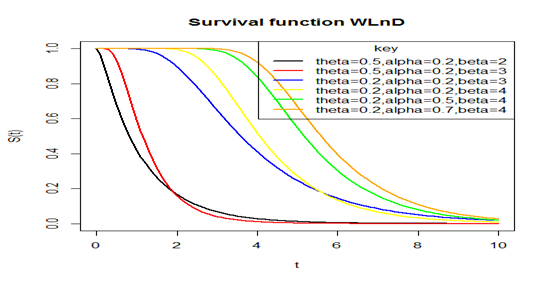Figure 3 Plot of the survival function of the WLnD for different parameter values.

The Hazard function

Hazard function as the name implies is also called risk function, it gives us the probability that a component will fail or die for an interval of time. The hazard function is defined mathematically as;

$h\left(x\right)=\frac{f\left(x\right)}{1-F\left(x\right)}=\frac{f\left(x\right)}{S\left(x\right)}$  (2.3.3)

Taking f(x) and F(x) to be the pdf and cdf of the proposed Weibull-Lindley distribution given previously, we obtain the hazard function as:

$h\left(x\right)=\frac{\alpha \beta {\theta }^{2}\left(1+x\right){e}^{-\theta x}{\left\{-\mathrm{log}\left[1-\left[1+\frac{\theta x}{\theta +1}\right]{e}^{-\theta x}\right]\right\}}^{\beta -1}{e}^{-\alpha {\left\{-\mathrm{log}\left[1-\left[1+\frac{\theta x}{\theta +1}\right]{e}^{-\theta x}\right]\right\}}^{\beta }}}{\left(\theta +1\right)\left[1-\left[1+\frac{\theta x}{\theta +1}\right]{e}^{-\theta x}\right]\left(1-{e}^{-\alpha {\left\{-\mathrm{log}\left[1-\left[1+\frac{\theta x}{\theta +1}\right]{e}^{-\theta x}\right]\right\}}^{\beta }}\right)}$ (2.3.4)

The following is a plot of the hazard function at chosen parameter values in Figure 4.Figure 4 Plot of the hazard function of the WLnD for different parameter values.

Figure 4 above shows the behavior of hazard function of the WLnD and it means that the probability of failure for any Weibull-Lindley random variable increases as the time or age of the variable grows or increases, that is, as time goes, the probability of failure or death increases and becomes constant after some times.

Order statistics

Order statistics are used widely over the years for solving a huge set of problems such as in robust statistical estimation and detection of outliers, characterization of probability distributions and goodness of fit tests, entropy estimation, analyses of censored samples, reliability analysis, quality control and strength of materials. Suppose ${X}_{1},{X}_{2},......,{X}_{n}$  is a random sample from a distribution with pdf, f(x), and let ${X}_{1:n}<{X}_{2:n}<......<{X}_{a:n}$  denote the corresponding order statistic obtained from this sample. The pdf, ${f}_{a:n}\left(x\right)$  of the ${a}^{th}$  order statistic can be defined as;

${f}_{a:n}\left(x\right)=\frac{n!}{\left(a-1\right)!\left(n-a\right)!}\sum _{k=0}^{n-a}{\left(-1\right)}^{k}\left(\begin{array}{c}n-a\\ k\end{array}\right)f\left(x\right){F\left(x\right)}^{k+a-1}$  (2.4.1)

where f(x) and F(x) are the pdf and cdf of the Weibull-Lindley distribution respectively.

Using (2.1.3) and (2.1.4), the pdf of the ${a}^{th}$  order statistics ${X}_{a:n}$ , can be expressed from (2.4.1) as;

${f}_{a:n}\left(x\right)=\frac{n!}{\left(a-1\right)!\left(n-a\right)!}\sum _{k=0}^{n-a}{\left(-1\right)}^{k}\left(\begin{array}{c}n-a\\ k\end{array}\right)\left[\frac{\alpha \beta {\theta }^{2}\left(1+x\right){e}^{-\theta x}{\left\{-\mathrm{log}\left[1-\left[1+\frac{\theta x}{\theta +1}\right]{e}^{-\theta x}\right]\right\}}^{\beta -1}}{\left(\theta +1\right)\left[1-\left[1+\frac{\theta x}{\theta +1}\right]{e}^{-\theta x}\right]{e}^{\alpha {\left\{-\mathrm{log}\left[1-\left[1+\frac{\theta x}{\theta +1}\right]{e}^{-\theta x}\right]\right\}}^{\beta }}}\right]*{\left[{e}^{-\alpha {\left\{-\mathrm{log}\left[1-\left[1+\frac{\theta x}{\theta +1}\right]{e}^{-\theta x}\right]\right\}}^{\beta }}\right]}^{a+k-1}$  (2.4.2)

Hence, the pdf of the minimum order statistic ${X}_{\left(1\right)}$  and maximum order statistic ${X}_{\left(n\right)}$  of the WLnD are given by;

${f}_{1:n}\left(x\right)=n\sum _{k=0}^{n-1}{\left(-1\right)}^{k}\left(\begin{array}{c}n-1\\ k\end{array}\right)\left[\frac{\alpha \beta {\theta }^{2}\left(1+x\right){e}^{-\theta x}{\left\{-\mathrm{log}\left[1-\left[1+\frac{\theta x}{\theta +1}\right]{e}^{-\theta x}\right]\right\}}^{\beta -1}}{\left(\theta +1\right)\left[1-\left[1+\frac{\theta x}{\theta +1}\right]{e}^{-\theta x}\right]{e}^{\alpha {\left\{-\mathrm{log}\left[1-\left[1+\frac{\theta x}{\theta +1}\right]{e}^{-\theta x}\right]\right\}}^{\beta }}}\right]*{\left[{e}^{-\alpha {\left\{-\mathrm{log}\left[1-\left[1+\frac{\theta x}{\theta +1}\right]{e}^{-\theta x}\right]\right\}}^{\beta }}\right]}^{k}$  (2.4.3)

And

${f}_{n:n}\left(x\right)=n\left[\frac{\alpha \beta {\theta }^{2}\left(1+x\right){e}^{-\theta x}{\left\{-\mathrm{log}\left[1-\left[1+\frac{\theta x}{\theta +1}\right]{e}^{-\theta x}\right]\right\}}^{\beta -1}}{\left(\theta +1\right)\left[1-\left[1+\frac{\theta x}{\theta +1}\right]{e}^{-\theta x}\right]{e}^{\alpha {\left\{-\mathrm{log}\left[1-\left[1+\frac{\theta x}{\theta +1}\right]{e}^{-\theta x}\right]\right\}}^{\beta }}}\right]{\left[{e}^{-\alpha {\left\{-\mathrm{log}\left[1-\left[1+\frac{\theta x}{\theta +1}\right]{e}^{-\theta x}\right]\right\}}^{\beta }}\right]}^{n-1}$  (2.4.4)

,p>respectively.

Parameter estimation via maximum likelihood

Let ${X}_{1},{X}_{2},....,{X}_{n}$ > be a sample of size ‘n’ independently and identically distributed random variables from the WLnD with unknown parameters α, β and Ө defined previously. The pdf of the WLnD is given from (2.1.3) as

$f\left(x\right)=\frac{\alpha \beta {\theta }^{2}\left(1+x\right){e}^{-\theta x}{\left\{-\mathrm{log}\left[1-\left[1+\frac{\theta x}{\theta +1}\right]{e}^{-\theta x}\right]\right\}}^{\beta -1}{e}^{-\alpha {\left\{-\mathrm{log}\left[1-\left[1+\frac{\theta x}{\theta +1}\right]{e}^{-\theta x}\right]\right\}}^{\beta }}}{\left(\theta +1\right)\left[1-\left[1+\frac{\theta x}{\theta +1}\right]{e}^{-\theta x}\right]}$

The likelihood function is given by;

$L\left(\underset{_}{X}|\alpha ,\beta ,\theta \right)=\frac{{\left(\alpha {\beta }^{\alpha }{\theta }^{2}\right)}^{n}\prod _{i=1}^{n}\left\{\left(1+{x}_{i}\right){e}^{-\theta {x}_{i}}\right\}\prod _{i=1}^{n}{\left\{-\mathrm{log}\left[1-\left[1+\frac{\theta {x}_{i}}{\theta +1}\right]{e}^{-\theta {x}_{i}}\right]\right\}}^{\beta -1}}{{\left(\theta +1\right)}^{n}\prod _{i=1}^{n}\left\{1-\left[1+\frac{\theta {x}_{i}}{\theta +1}\right]{e}^{-\theta {x}_{i}}\right\}{e}^{\alpha \sum _{i=1}^{n}{\left\{-\mathrm{log}\left[1-\left[1+\frac{\theta {x}_{i}}{\theta +1}\right]{e}^{-\theta {x}_{i}}\right]\right\}}^{\beta }}}$  (2.5.1)

Let the log-likelihood function, $l=\mathrm{log}L\left(\underset{_}{X}|\alpha ,\beta ,\theta \right)$ , therefore

$l=n\mathrm{log}\alpha +n\mathrm{log}\beta +2n\mathrm{log}\theta -n\mathrm{log}\left(\theta +1\right)+\sum _{i=1}^{n}\mathrm{log}\left(1+{x}_{i}\right)-\theta \sum _{i=1}^{n}{x}_{i}+\left(\beta -1\right)\sum _{i=1}^{n}\mathrm{log}\left\{-\mathrm{log}\left[1-\left[1+\frac{\theta {x}_{i}}{\theta +1}\right]{e}^{-\theta {x}_{i}}\right]\right\}$

$-\sum _{i=1}^{n}\mathrm{log}\left(\left[1-\left[1+\frac{\theta {x}_{i}}{\theta +1}\right]{e}^{-\theta {x}_{i}}\right]\right)-\alpha \sum _{i=1}^{n}{\left\{-\mathrm{log}\left[1-\left[1+\frac{\theta {x}_{i}}{\theta +1}\right]{e}^{-\theta {x}_{i}}\right]\right\}}^{\beta }$  (2.5.2)

Differentiating $l$  partially with respect to α, β and Ө respectively gives;

$\frac{\partial l}{\partial \alpha }=\frac{n}{\alpha }-\sum _{i=1}^{n}{\left\{-\mathrm{log}\left[1-\left[1+\frac{\theta {x}_{i}}{\theta +1}\right]{e}^{-\theta {x}_{i}}\right]\right\}}^{\beta }$  (2.5.3)

$\frac{\partial l}{\partial \beta }=\frac{n}{\beta }+\sum _{i=1}^{n}\mathrm{log}\left\{-\mathrm{log}\left[1-\left[1+\frac{\theta {x}_{i}}{\theta +1}\right]{e}^{-\theta {x}_{i}}\right]\right\}-\alpha \sum _{i=1}^{n}{\left\{-\mathrm{log}\left[1-\left[1+\frac{\theta {x}_{i}}{\theta +1}\right]{e}^{-\theta {x}_{i}}\right]\right\}}^{\beta }\left\{-\mathrm{log}\left[1-\left[1+\frac{\theta {x}_{i}}{\theta +1}\right]{e}^{-\theta {x}_{i}}\right]\right\}$  (2.5.4)

$\frac{\partial l}{\partial \theta }=\frac{2n}{\theta }-\frac{n}{\theta +1}-\sum _{i=1}^{n}{x}_{i}+\left(\beta -1\right)\sum _{i=1}^{n}\left\{\frac{{x}_{i}{e}^{-\theta {x}_{i}}\left[\frac{1}{{\left(\theta +1\right)}^{2}}-\frac{\theta {x}_{i}+\theta +1}{\theta +1}\right]}{\left\{-\mathrm{log}\left[1-\left[1+\frac{\theta {x}_{i}}{\theta +1}\right]{e}^{-\theta {x}_{i}}\right]\right\}\left\{\left[1-\left[1+\frac{\theta {x}_{i}}{\theta +1}\right]{e}^{-\theta {x}_{i}}\right]\right\}}\right\}-\sum _{i=1}^{n}\left\{\frac{{x}_{i}{e}^{-\theta {x}_{i}}\left[\frac{1}{{\left(\theta +1\right)}^{2}}-\frac{\theta {x}_{i}+\theta +1}{\theta +1}\right]}{\left(\left[1-\left[1+\frac{\theta {x}_{i}}{\theta +1}\right]{e}^{-\theta {x}_{i}}\right]\right)}\right\}$

$+\alpha \beta \sum _{i=1}^{n}\left\{\frac{{x}_{i}{e}^{-\theta {x}_{i}}\left[\frac{1}{{\left(\theta +1\right)}^{2}}-\frac{\theta {x}_{i}+\theta +1}{\theta +1}\right]{\left\{-\mathrm{log}\left[1-\left[1+\frac{\theta {x}_{i}}{\theta +1}\right]{e}^{-\theta {x}_{i}}\right]\right\}}^{\beta -1}}{\left[1-\left[1+\frac{\theta {x}_{i}}{\theta +1}\right]{e}^{-\theta {x}_{i}}\right]}\right\}$  (2.5.5)

Equating equations (2.5.3), (2.5.4) and (2.5.5) to zero and solving for the solution of the non-linear system of equations will give us the maximum likelihood estimates of parameters $\alpha ,\beta &\theta$  respectively. However, the above equations cannot be solved manually due to their complexity unless numerically with the help of statistically inclined computer programs like Python, R, SAS, etc., when data sets are available.

# Results and discussions

This section presents four datasets, their descriptive statistics, graphics and applications to some selected extensions of the Lindley distribution including the classical Lindley distribution. We have compared the performance of the Weibull-Lindley distribution (WLlD) to some families of Lindley distribution such as Lomax-Lindley distribution (LLlD), Two-parameter Lindley distribution (TPLlD), Transmuted Lindley distribution (TLlD) and the Lindley distribution (LlD).

Data sets and their nature

In this section, four different datasets and their summary are presented for fitting the above listed distributions. The available data sets I, II, III, and IV and their respective summary statistics are provided in Table 1–5 respectively as follows;

 Parameters n Minimum Median Mean Maximum Variance Skewness Kurtosis Values 20 1.1 1.475 1.7 2.05 1.9 4.1 0.4958 1.8625 7.1854

Table 1 Summary statistics for dataset I

 Parameters n Minimum Median Mean Maximum Variance Skewness Kurtosis Values 20 40 86.75 119 140.8 113.45 165 1280.892 -0.3552 -0.89

Table 2 Summary statistics for dataset II

 Parameters n Minimum Median Mean Maximum Variance Skewness Kurtosis Data set I 63 0.55 1.375 1.59 1.685 1.507 2.24 0.105 -0.8786 3.9238

Table 3 Summary Statistics for data set III

 Parameters n Minimum Median Mean Maximum Variance Skewness Kurtosis Values 59 4.1 8.45 10.6 16.85 13.49 39.2 64.8266 1.6083 2.256

Table 4 Descriptive statistics for dataset IV

 Parameter estimates ƖƖ=(log-likelihood value) AIC A* W* K-S P-Value Ranks (K-S) 0.5842 16.0004 38.0009 0.2483 0.0428 0.167 0.6324 1 1.2337 3.8112 1.1589 24.9726 53.9452 0.6295 0.1063 0.3113 0.0414 2 -0.9888 0.8926 27.2805 58.561 0.6401 0.108 0.2885 0.0716 3 9.7008 0.8162 30.2496 62.4991 0.6758 0.1141 0.3911 0.0044 4 0.361 29.8421 65.6841 0.6552 0.1107 0.416 0.002 5 9.6021 2.4483

Table 5 The statistics ll, AIC, A*, W* and K-S for the fitted models to the first dataset

Dataset I: This dataset represents the lifetime’s data relating to relief times (in minutes) of 20 patients receiving an analgesic and reported by Gross et al.,34 and has been used by Shanker et al.,35 Table 1.

Dataset II: This data represent the survival times in weeks for male rats Lawless et al.,36 (Table 2).

Data set III: This data set represents the strength of 1.5cm glass fibers initially collected by members of staff at the UK national laboratory. It has been used by Bourguignon et al.,18 Afify et al.,37  Barreto Souza et al.,38 Oguntunde et al.,39 Ieren  et al.,40 as well as Smith et al.,41 Its summary is given as follows: (Table 3).

Dataset IV: This dataset represents 59 observations of the monthly actual taxes revenue in Egypt (in 1,000 million Egyptian pounds) between January 2006 and November 2010. The data has been previously used by Owoloko et al.42 The descriptive statistics for this data are as follows:

We also provide some histograms and densities for the three data sets as shown in Figure 5–8 below respectively (Table 4).

From the summary statistics of the four data sets, we found that data sets I and IV are positively skewed, while II is a bit negatively skewed or approximately normal and III is negatively skewed. Also, data sets I, III and IV have higher kurtosis while II have low level or degree of peakness.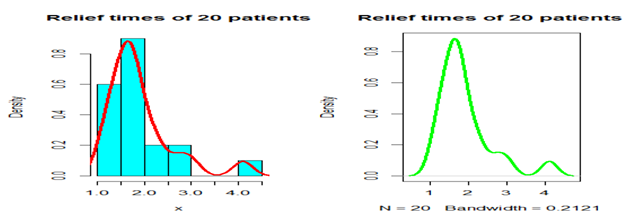Figure 5 Histogram and density plot for the Relief times of 20 patients (Data set I).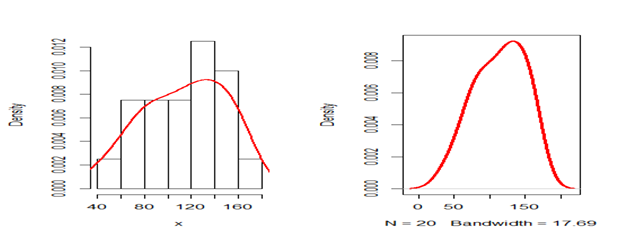Figure 6 Histogram and density plot for the survival times in weeks for male rats (Data set II).Figure 7 A histogram and density plot for the strength of 1.5cm glass fibres (Data set III).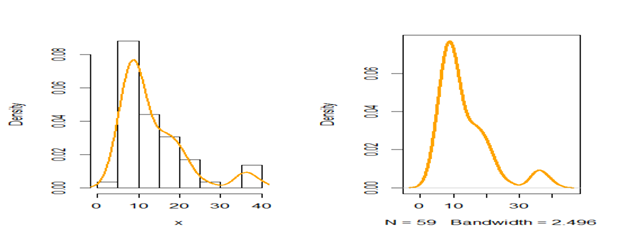Figure 8 A histogram and density plot for the monthly actual taxes revenue in Egypt (Data set IV).

Analysis of data

These four different datasets presented above were used to fit all the above listed Lindley distributions by applying the formulas of the test statistics in section 2 in order to get the best fitted model and the results are presented as follow in the four tables for each dataset below: (Table 5).

From Table 5, the values of the parameter MLEs and the corresponding values of ƖƖ, AIC, A*, W* and K-S for each model show that the Weibull-Lindley distribution (WLlD) has better performance compared to the other four models namely: Lomax-Lindley distribution (LLlD), Two-parameter Lindley distribution (TPLlD), Transmuted Lindley distribution (TLlD) and the Lindley distribution (LlD) and hence becomes the best fitted distribution based the data set I (Table 6).

 Parameter estimates ƖƖ=(log-likelihood value) AIC A* W* K-S P-Value Ranks (K-S) 0.0278 106.6467 219.2935 0.4311 0.062 0.3225 0.0313 1 1.6862 1.0152 0.0198 112.8891 231.7781 0.5199 0.076 0.3772 0.0068 2 1.9445 2.8037 0.0371 128.3464 260.6929 0.4103 0.0586 0.6941 8.57E-09 3 0.3054 1.9987 4442.078 8888.156 NaN NaN 1 <2.2e-16 5 0.6326 2.2161 4926.226 9854.452 NaN NaN 1 <2.2e-16 4

Table 6 The statistics ll, AIC, A*, W* and K-S for the fitted models to the second dataset

Again the results in Table 6 above shows that the Weibull-Lindley distribution (WLlD) fits the second dataset better than the other four models (LLlD, TPLlD, TLlD and LlD) because the values of the statistics; AIC, A*, W* and K-S are smaller for the WLlD than the other models and therefore it is considered as the best fitted distribution based the data set II (Table 7).

 Distributions Parameter estimates ƖƖ=(log-likelihood value) AIC A* W* K-S P-Value Ranks (K-S) 1.4523 34.1708 74.3416 4.2254 0.7768 0.224 0.0033 1 7.6251 1.7248 0.361 81.4714 168.9428 3.074 0.5619 0.3213 3.60E-06 2 9.6021 2.4483 1.391 63.8482 131.6963 3.1901 0.5833 0.3413 6.70E-07 3 -0.9937 1.2155 71.0355 146.071 3.1414 0.5744 0.3427 5.90E-07 4 9.2573 0.9957 82.5853 167.1706 3.0788 0.563 0.3885 8.20E-09 5

Table 7 The statistics ll, AIC, A*, W* and K-S for the fitted models to the third dataset

The results from Table 7 also agrees with the previous results that the WLlD is more flexible compared to the three other models this also agrees with the fact that generalizing any continuous distribution provides a compound distribution with at least better fit than the classical distribution (i.e Lindley) irrespective of the nature of the data used provide it is asymmetry Table 8.

 Distributions Parameter estimates ƖƖ=(log-likelihood value) AIC A* W* K-S P-Value Ranks (K-S) 0.3767 199.8163 405.6327 0.7927 0.1329 0.1597 0.0986 1 8.5414 0.8256 0.0809 201.0534 408.1067 1.127 0.1849 0.1558 0.1139 2 6.6768 3.1649 0.1429 199.6626 403.3251 1.4251 0.229 0.1676 0.0728 5 -0.4154 0.1618 199.324 402.648 1.2489 0.2007 0.2084 0.0119 3 4.938 0.1361 200.6599 403.3198 1.2999 0.2087 0.1844 0.0361 4

Table 8 The statistics ll, AIC, A*, W* and K-S for the fitted models to the fourth dataset

Lastly, our results in Table 8 provides the same results as obtained in the above previous tables with the Weibull-Lindley distribution performing better than the other three distributions considered in this study.

The following figures displayed the histogram and estimated densities of the fitted models for the four real life data sets used in this study.

From the estimated density plots in Figures 9 we can observe that though there is no big difference between the performance of the other four models, it is very clear that the performance of the Weibull-Lindley distribution (WLlD) remains the best and consistent irrespective of the nature the datasets as compared to the Lomax-Lindley distribution (LLlD), Two-parameter Lindley distribution (TPLlD), Transmuted Lindley distribution (TLlD) and the Lindley distribution (LlD).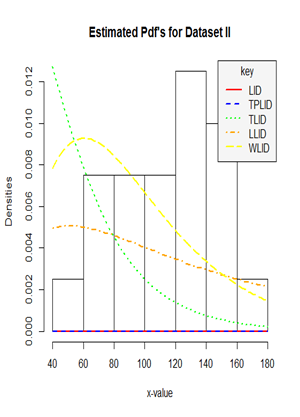Figure 9 Histogram and estimated densities of the Weibull-Lindley distribution (WLlD), Lomax-Lindley distribution (LLlD), Two-parameter Lindley distribution (TPLlD), Transmuted Lindley distribution (TLlD) and the Lindley distribution (LlD) for the four real life datasets (dataset I, II, III and IV).

Furthermore, the performance of the Weibull-Lindley could be attributed to the fact that the Weibull-Lindley distribution is heavy-tailed and highly skewed to the right with excellent flexibility which allows it to take various shapes depending on the parameter values and it also exhibit some degree of kurtosis all of which are features of the four datasets used in this research, hence, the Weibull-Lindley distribution will be more appropriate for lifetime datasets which are positively skewed with a higher degree of peakness as well as those that are approximately normal with observations above zero.

Hence, having demonstrated earlier in Tables 5–8, we have a similar conclusion based on figure 3-5 that the Weibull-Lindley distribution has a better fit for the four data sets considered in this study.

# Summary conclusion

This article proposed a new distribution called Weibull-Lindley distribution. The Mathematical and Statistical properties of the new distribution have been derived and studied extensively. Some of its properties with graphical analysis and discussion on their usefulness and applications were also considered. The model parameters were estimated using maximum likelihood method and we have a conclusion based on our applications of the model to four real life datasets that the new distribution (WLnD) has a better fit compared to the other four already existing models considered in this study.

None.

# Conflict of interest

Author declares that there is no conflicts of interest.